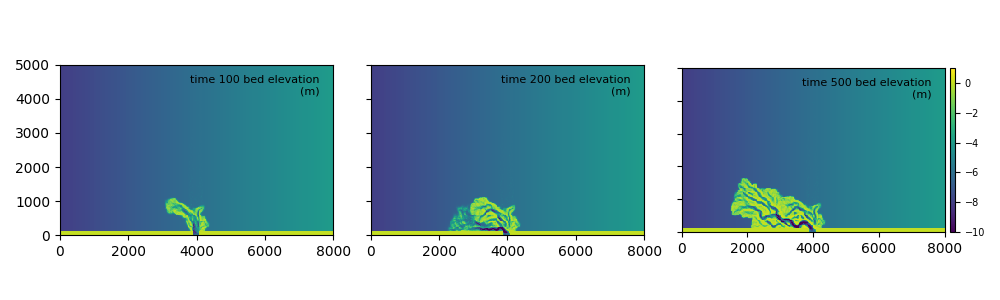# pyDeltaRCM Model Subclassing for customization

A component of my postdoctoral research award includes improving flexibility, accessibility, and reproducibility of delta numerical models. In particular, my efforts revolve around the pyDeltaRCM numerical delta model. In this post I explain how the model flexibility is maintained, and show an example of how to use the model to test a scientific research hypothesis.

The pyDeltaRCM.DeltaModel is designed for flexibility and extension by users, and to support arbitrary and imaginative changes to the model routine. For example, one can easily extend the model to include additional delta controls (such as vegetation or permafrost development), or modify the model domain boundary conditions (such as imposing a sloped receiving basin). This flexibility is achieved by “subclassing” the main model within pyDeltaRCM, the DeltaModel. In subclassing, we create a custom model object, and utilize “hooks” in the model to achieve our desired modifications.

For this example, we consider the case where we are a researcher seeking to explore the effects of a receiving basin that is sloped perpendicular to the channel outlet. This researcher asks: does this sloped basin cause channels to steer towards the deeper water, where compensation is higher?

Subclassing is a standard concept in object-oriented programming, whereby a subclass obtains all the functionality of the parent object, and then adds/modifies existing functionality of the parent, to create a new class of object (i.e., the subclass). To subclass the DeltaModel we simply create a new Python object class, which inherits from the model class:

import pyDeltaRCM

class SlightSlopeModel(pyDeltaRCM.DeltaModel):
def __init__(self, input_file):
super().__init__(input_file)  # inherit base DeltaModel methods


Hooks are methods in the model sequence that, do nothing by default, but can be augmented to provide arbitrary desired behavior in the model. Hooks are been integrated throughout the DeltaModel initialization and update() sequences, to allow the users to achieve complex behavior at various times throughout the default model sequence. For example, after_init() is a hook which occurs after the default model initialization is complete. To utilize the hooks, we simply define a method in our subclass with the name corresponding to the hook we want to augment, so to achieve the desired behavior.

In our example, this amounts to defining a method matching the after_init() hook, wherein we modify the bed elevation (eta) of the model to slope perpendicularly to the channel. L0 is the channel inlet length (in number of cells), where we want to leave the bed elevation unchanged. So, we first compute a line with specified slope, covering the width of the model domain (i.e., the direction perpendicular to the channel). Then, we create a grid from this line with the np.tile function; on this same line, we subtract the medial bed elevation of the line from the entire grid, so to make the medial elevation of the line 0. In this way, when we add the grid to the existing bed elevation in the final line, the bed elevation at the channel is approximately unchanged.

class SlightSlopeModel(pyDeltaRCM.DeltaModel):
def __init__(self, input_file):
super().__init__(input_file) # inherit base DeltaModel methods

def after_init(self):
"""Called at end of initialize."""
_slope = 0.0005
lin = _slope * np.arange(0, self.Width, step=self.dx)
grid = np.tile(lin, (self.L - self.L0, 1)) - ((_slope*self.Width)/2)
self.eta[self.L0:, :] = self.eta[self.L0:, :] + grid



Now, with this subclass, during the initializing of a SlightSlopeModel instance, our implementation of after_init() will be called and the bed elevation will be adjusted accordingly. Coupling this custom model subclass with the a simple YAML configration file (reproduced at the end of this post), we investigate the effect of a sloped receiving basin.While this is not a robust scientific study, this simple modification seems to support the notion that the channels are steered towards deeper water where there is higher accommodation. This is a simple demonstration of how to subclass the pyDeltaRCM model and create a custom model implementation, but it is far from an exhaustive example. There are many more hooks in the model, described thoroughly in the model documentation.

YAML configuration file:

Length: 5000.
Width: 8000.
dx: 25
Np_water: 1000
Np_sed: 1000